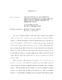## Measurement of Atmospheric Neutrino Oscillation Parameters Using Three Years of IceCube-DeepCore DataThe story of neutrinos began in 1930 when Pauli proposed a hypothesized particle as a desperate remedy" to rescue quantum theory. Although Pauli was pessimistic about the detectability of his new particle, Reins and Cowan first discovered (anti) neutrinos in 1956. Soon after, neutrinos became a puzzle for particle physicists due to a persistent deficit in observed rates by multiple experiments. This mystery was partly answered by Pontecorvo who first proposed the idea of neutrino oscillations in 1957. In 1998, the Super-Kamiokande (SK) collaboration provided the first definitive evidence of neutrino oscillations, for which both the SK and the Sudbury Neutrino Observatory (SNO) collaborations were awarded the Nobel Prize in Physics 2015. While measuring oscillation parameters has long been a focus for numerous neutrino experiments, the IceCube Neutrino Observatory with DeepCore provides a unique window to measure atmospheric oscillation parameters. With an effective volume $\sim$ 300 times larger than SK, DeepCore can detect atmospheric neutrinos between a few and 100 GeV. In addition, IceCube acts as a thick veto shield for DeepCore to better identify atmospheric muon backgrounds. Given that the amplitude of atmospheric neutrino oscillations is expected to be maximal at $\sim$ 25 GeV, IceCube-DeepCore is well suited for studying atmospheric neutrino oscillations by probing this energy window for the first time. Using three years of IceCube-DeepCore data from 2012 to 2014, this work measures atmospheric neutrino oscillation parameters from the disappearance of muon neutrinos. The standard three neutrino mixing and matter effect due to Earth are considered. Under the assumption of a unitary mixing matrix, a binned analysis using a modified $\chi^2$ is performed, and sixteen systematics are taken into account. Preferring a normal neutrino mass ordering, this analysis measures the mass squared difference, $\Delta$m$^2_{23} = 2.55^{+0.12}_{-0.11} \times 10^{-3}$ eV$^2$, and the mixing angle, sin$^2 \theta_{23} = 0.58^{+0.04}_{-0.13}$. The measurement from this work is comparable to the latest measurements from other long baseline neutrino experiments.